# SAT II Math I : Real and Complex Numbers

## Example Questions

2 Next →

### Example Question #41 : Number Theory

Which of the following choices gives a sixth root of sixty-four?

All of these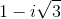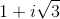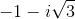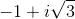All of these

Explanation:

Letbe a sixth root of 64. The question is to find a solution of the equation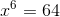.

Subtracting 64 from both sides, this equation becomes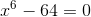64 is a perfect square (of 8) The binomial at left can be factored first as the difference of two squares: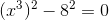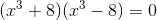8 is a perfect cube (of 2), so the two binomials can be factored as the sum and difference, respectively, of two cubes: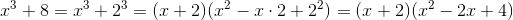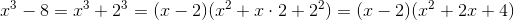The equation therefore becomes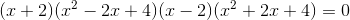.

By the Zero Product Principle, one of these factors must be equal to 0.

If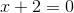, then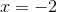; if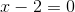, then. Therefore,and 2 are sixth roots of 64. However, these are not choices, so we examine the other polynomials for their zeroes.

If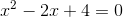, then, setting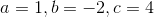in the following quadratic formula: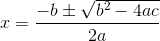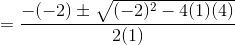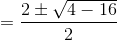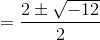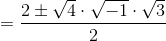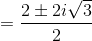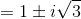If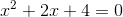, then, setting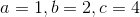in the quadratic formula: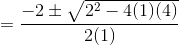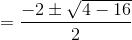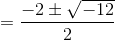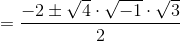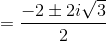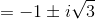Therefore, the set of sixth roots of 64 is.

All four choices appear in this set.

### Example Question #41 : Number Theory

Letandbe complex numbers.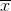and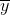denote their complex conjugates.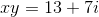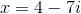Evaluate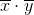.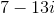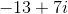None of these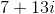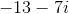None of these

Explanation:

Knowing the actual values ofandis not necessary to solve this problem. The product of the complex conjugates of two numbers is equal to the complex conjugate of the product of the numbers; that is,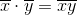, so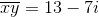, and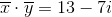,

which is not among the choices.

### Example Question #41 : Sat Subject Test In Math Idenotes the complex conjugate of.

If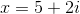, then evaluate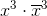.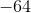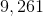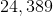None of these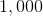Explanation:

Applying the Power of a Product Rule: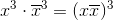The complex conjugate of an imaginary number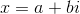is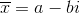; the product of the two is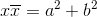, so, setting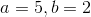in the above pattern: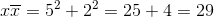Consequently,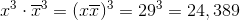2 Next →

### All SAT II Math I Resources